### PV Function – Present Value in Excel, VBA, Google Sheets

This tutorial demonstrates how to use the Excel PV Function in Excel to calculate the present value of an investment.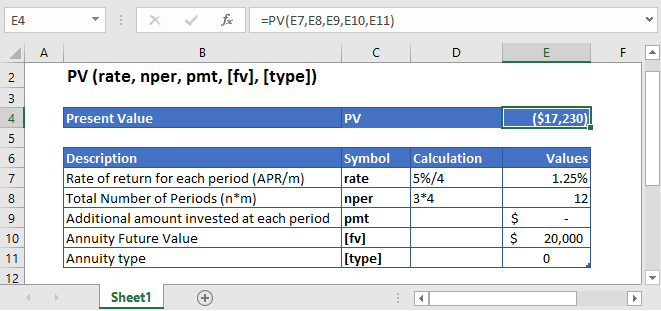## PV Function Overview

The PV Function Calculates the present value.

To use the PV Excel Worksheet Function, select a cell and type: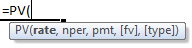(Notice how the formula inputs appear)

### PV Function Syntax and Inputs:

`=PV(rate,nper,pmt,fv,type)`

rate – The interest rate for each period.

nper – It’s the total number of payment periods.

pmt – It’s the payment per period.

fv – OPTIONAL: The Future Value of the investment or loan at the end of the number of payment periods.

Type – OPTIONAL: The type argument shows when the payments are made, either at the end of the period by 0 or at the start of the period by 1. Its default value is 0.

AutoMacro - VBA Code Generator

## What Is Present Value?

Present Value is the current value of the money that’s going to be received in the future with a particular rate of return.  It’s based on a basic financial concept that the current amount of money is worth more than the same amount of money which will be received in the future.

The Present Value is calculated as: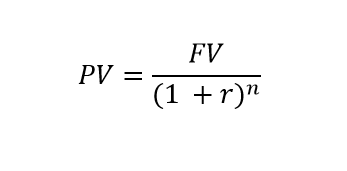Where:

FV = Future Value

r = Rate of Return

n = Number of periods

## What Is Excel PV Function?

PV is one of the financial functions that calculates the present value of either an investment or a loan, based on a consistent number of payments, which are going to be made in the future, at a constant interest rate.

### Calculate Present Value of an Investment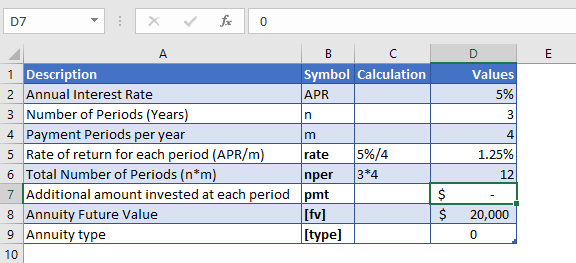Let’s find out the present value on an investment, which will pay \$20,000 at the end of its three years investment period. The annual interest rate on the initial investment is 5% which is compounded quarterly.

The annual interest rate is converted into quarterly interest as

Quarterly Interest Rate – 5% (annual interest rate) / 4 (quarters per year) = 1.25%

and the number of payments per period is converted into the quarterly payments by

NPER – 3 (years) * 4 (quarters per year) = 12

No regular payments are being made, so the value of pmt argument is

PMT = 0

The formula used for the calculation of present value is:

`=PV(D7,D8,D9,D10,D11)`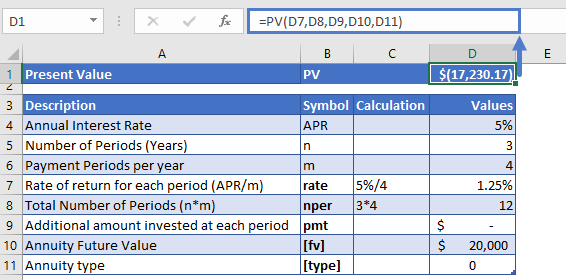The present value of the investment is

PV = -\$17,230

The returned present value is negative as it represents outgoing payment.

### Calculate Present Value of an Insurance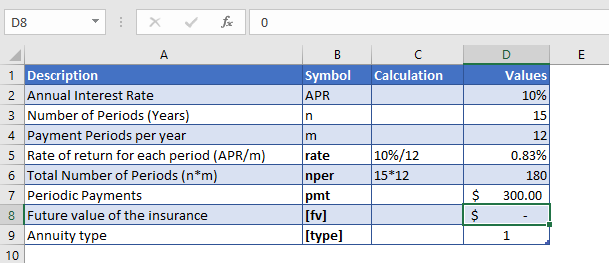Suppose insurance is bought, in which the regular payments of \$300 have to be paid at the start of every month to the insurance company for the next 15 years. The interest earned on this insurance is 10% per year but it will be compounded monthly. So, the present value of insurance is calculated.

As the payments are made monthly, the annual interest rate is converted into monthly interest by

Monthly Interest Rate – 10% (annual interest rate) / 12 (months per year) = 0.83%

and the number of payments per period is converted into the monthly number of payments by

NPER – 15 (years) * 12 (months per year) = 180

The formula used for the calculation is:

`=PV(D7,D8,D9,D10,D11)`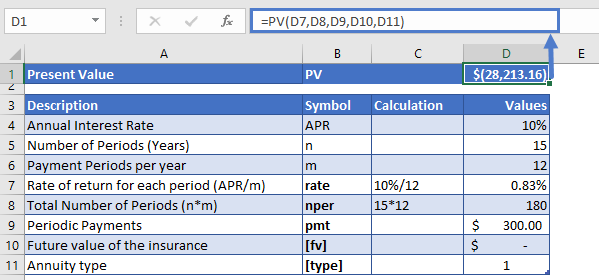The present value of the investment is

PV = -\$28,213

## PV in Google Sheets

All of the above examples work exactly the same in Google Sheets as in Excel.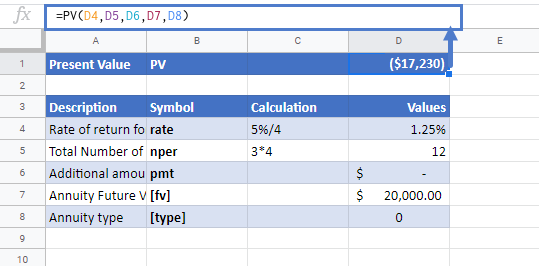## Additional Notes

Use the PV Function to calculate the Present Value of an investment.
Investopedia defines present value as: The current worth of a future sum of money or stream of cash flows given a specified rate of return.

Make sure the units of nper and rate are consistent, e.g., in case of monthly interest rate the number of periods of investment should also be in months.

In the Financial Functions the cash outflows, such as deposits, are represented by negative numbers and the cash inflows, such as dividends, are represented by positive numbers.

## PV Examples in VBA

You can also use the PV function in VBA. Type:
`application.worksheetfunction.pv(rate,nper,pmt,fv,type)`
For the function arguments (rate, etc.), you can either enter them directly into the function, or define variables to use instead.

Return to the List of all Functions in Excel

## Excel Practice Worksheet

Practice Excel functions and formulas with our 100% free practice worksheets!

• Automatically Graded Exercises
• Learn Excel, Inside Excel!